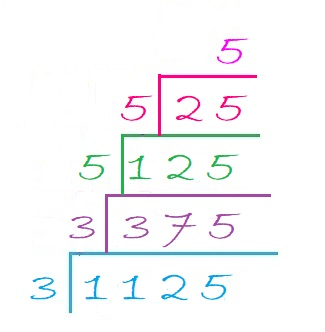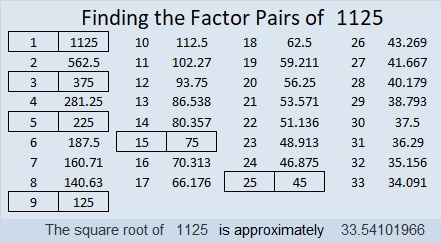# 1125 May I Interest You In a Little Cake?

1 + 1 + 2 + 5 = 9, so 1125 can be evenly divided by both 3 and 9.
1125 can also be evenly divided by 5 because the last digit can be divided by 5.
1125 can be evenly divided by 25 because the last two digits can be divided by 25.
1125 can be evenly divided by 125 because the last three digits can be divided by 125.

May I interest you in a little cake? Although I’ve put many factor trees on this website, I actually prefer making factor cakes. I like them because all the prime factors can easily be found IN ORDER on the outside of the cake. The more layers the factor cake has, the more I like it. Here is THE factor cake for 1125:As you can see, the prime factorization of 1125 is 3 × 3 × 5 × 5 × 5. All of its prime factors are written nicely in order. None of them are hiding anyplace like they might in a factor tree.

Here are some facts about the number 1125:

• 1125 is a composite number.
• Prime factorization: 1125 = 3 × 3 × 5 × 5 × 5, which can be written 1125 = 3² × 5³
• The exponents in the prime factorization are 2 and 3. Adding one to each and multiplying we get (2 + 1)(3 + 1) = 3 × 4 = 12. Therefore 1125 has exactly 12 factors.
• Factors of 1125: 1, 3, 5, 9, 15, 25, 45, 75, 125, 225, 375, 1125
• Factor pairs: 1125 = 1 × 1125, 3 × 375, 5 × 225, 9 × 125, 15 × 75, or 25 × 45
• Taking the factor pair with the largest square number factor, we get √1125 = (√225)(√5) = 15√5 ≈ 33.541021125 is the hypotenuse of three Pythagorean triples:
675-900-1125 which is (3-4-5) times 225,
315-1080-1125 which is (7-24-25) times 45, and
396-1053-1125 which is 9 times (44-117-125)

1125 looks interesting to me when it is written in these bases:
It’s 5A5 in BASE 14 (A is 10 base 10) because 5(14²) + 10(14) + 5(1) = 1125
500 in BASE 15 because 5(15²) = 1125, and
it’s 3F3 in BASE 17 (F is 15 base 10) because 3(17²) + 15(17) + 3(1) = 1125